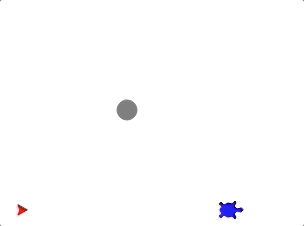# 10.1. Introduction¶

Recall how we used a turtle object to draw a square:

Example

 ```1 2 3 4 5 6 7 8``` ```import turtle bob = turtle.Turtle() side_length = 75 for side in range(4): bob.forward(side_length) bob.left(90) ```

To draw a square, we need to create a turtle and specify the length of one side. The `for` loop runs, and our turtle does its job.What if we have several different turtles on the screen, and we want each one to draw a square? We could copy and paste the `for` loop once for each turtle, but we would need to update the `forward` and `left` statements to match the turtle names.

Example

 ``` 1 2 3 4 5 6 7 8 9 10 11 12 13 14 15 16 17 18 19 20 21 22``` ```import turtle bob = turtle.Turtle() wonder = turtle.Turtle() im_really_a_tortoise = turtle.Turtle() # Turtle color, shape, speed, and location code... side_length = 50 for side in range(4): bob.forward(side_length) bob.left(90) side_length = 75 for side in range(4): wonder.forward(side_length) wonder.left(90) side_length = 100 for side in range(4): im_really_a_tortoise.forward(side_length) im_really_a_tortoise.left(90) ```

This is inefficient!Instead of copying, pasting, and then editing the loop once for each turtle, it would be nice if we could use the SAME lines of code over and over again.

Functions allow us to do this!

## 10.1.1. What is a Function?¶

A function is a reusable piece of code, and it consists of a series of statements that belong together. Like variables, we assign functions names. By calling a function name, we tell Python to run that block of code when we need it.

You used a function when you wrote your first Python program:

```print('Hello, World!')
```

To display information in the console, the `print` function follows a detailed set of instructions to convert the values inside the parentheses to symbols on the screen. We do not see these steps, but we execute them every time we use the function.

For the three-turtle example above, imagine we create a function named `draw_square`. We could put the `for` loop inside this function, and then run the function once for each turtle. This allows us to use the same lines of code for each turtle instead of writing multiple copies of the instructions.

Example

 ``` 1 2 3 4 5 6 7 8 9 10 11 12``` ```import turtle bob = turtle.Turtle() wonder = turtle.Turtle() im_really_a_tortoise = turtle.Turtle() # Turtle color, shape, speed, and location code... # draw_square function definition ... draw_square(bob, 50) draw_square(wonder, 75) draw_square(im_really_a_tortoise, 100) ```

Each time we run the `draw_square` function, we tell Python which turtle to use as well as the value for `side_length`.

When you wrote your `'Hello, World!'` program, we skipped the full explanation of how functions work. You are now ready to learn those details, as well as how functions can be used and how to create your own.

## 10.1.2. Function Control Flow¶

The control flow of a program is the order in which the statements are executed. Normal control flow runs from the top to the bottom of a file. However, we have seen how conditionals and loops alter the flow of a program by adding branches or repeated steps in the top-to-bottom running of the code.

Functions create blocks of code that remain separate from the main flow of a program. Think of these like complete, but separate, programs that sit off to the side of the main branch. When we call a function, the main program passes control to the separate branch. When the function completes its job, it returns control to the main program at the point just after the call was made.

Once a program finishes with a conditional or a loop, that code is done. The statements in a function, however, can be used again and again at different points in the program.